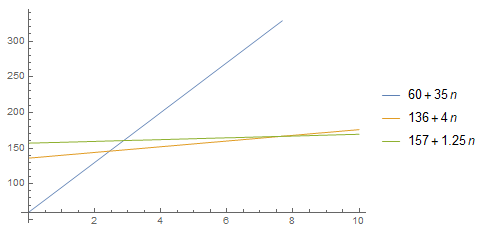# CSAPP第五章读书笔记

CSAPP第五章的读书笔记。

## 5.2 表示程序性能

${\bf N{\scriptsize OTE}}.$ 这个概念和算法的时间复杂度无关，因为 CPE 关注的是过程在给定的向量长度上的执行性能。

### 练习题 5.2• 当 $x \in [0..2]$ 时，$60 + 35n$ 最快；
• 当 $x \in [3..7]$ 时，$136 + 4n$ 最快；
• 当 $x \geq 8$ 时，$157 + 1.25n$ 最快。

## 5.4 消除循环的低效率

 1  for(int i = 0; i < strlen(s); i++); 

 1 2  int len = strlen(s); for(int i = 0; i < len; i++); 

A.1919090
B.9119090
C.119090

## 5.5 减少过程调用

${\bf N{\scriptsize OTE}}.$ 书中实例代码的安全性问题：

combine3 之前的版本，循环过程是按如下方式进行的：

 1 2 3 4 5  for(i = 0; i < length; i++) { data_t val; get_vec_element(v, i, &val); *dest = *dest OP val; } 

## 5.6 消除不必要的内存引用

 1 2  combine3(v, get_vec_start(v) + 2); combine4(v, get_vec_start(v) + 2); 

combine3$[2,3,5]$$[2,3,1]$$[2,3,2]$$[2,3,6]$$[2,3,36]$$[2,3,36] combine4[2,3,5]$$[2,3,5]$$[2,3,5]$$[2,3,5]$$[2,3,5]$$[2,3,30]$